# Measurement

 1 Introduction to Measurement 2 Measurement 3 Perimeter 4 Area 5 Solid Shapes 6 Surface Area 7 Volume 8 Time 9 FREE Worksheets of Measurement

## Introduction

The word “measurement” is derived from the Greek word "metron," which means a limited proportion. This word also finds its roots in the words "moon" and "month," possibly because astronomical objects were among the first methods to measure time.

We can measure the length, weight, and capacity (volume) of any given object. Let us

• learn how to measure the area and perimeter of plane shapes.
• explore solid shapes and learn how to measure their surface areas and volumes.

You can go ahead and explore all important topics in Measurement by selecting the topics from this list below:

 Measurements Surface Area Perimeter Volume Area Time Solid Shapes

## Measurement

Measurement involves measuring or quantifying the length, weight, and capacity of something. There are two main "Systems of Measurement": Metric and US Standard.

The table given below summarizes the frequently used units of measurement for length, weight, and capacity in both the Systems of Measurement.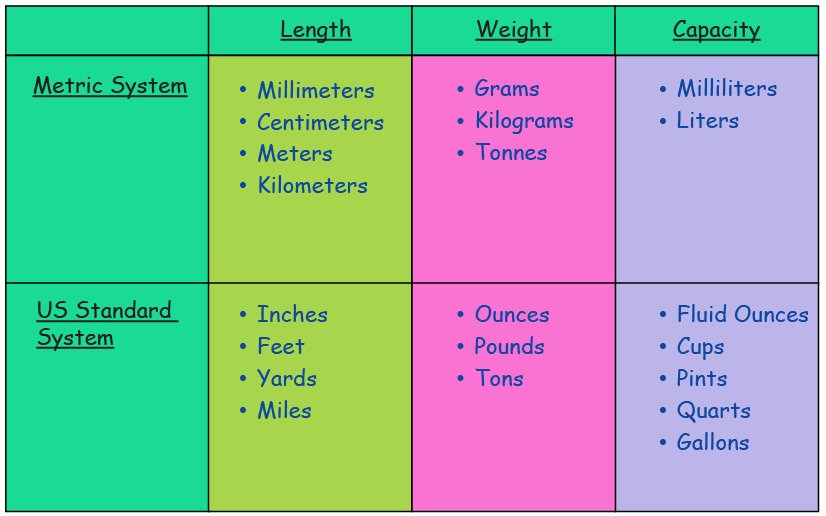Measurements are made by comparing a quantity with a standard unit. In this section, you will learn about Measuring Length, Measuring Weight, and Measuring Capacity.

## Perimeter

The total length of the boundary of a closed two-dimensional shape is called its perimeter.

Example: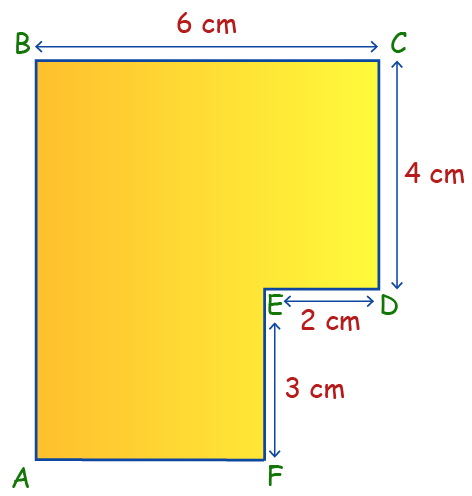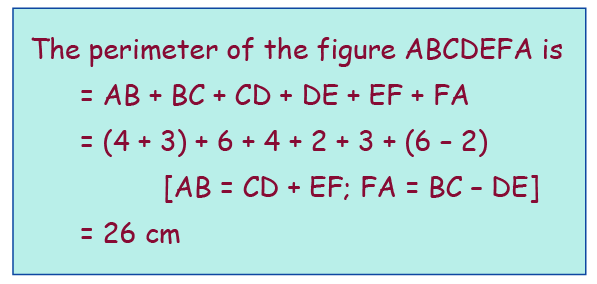We now know that Perimeter is the length of the boundary of a 2-dimensional figure. Let us now understand how to determine the Perimeter of a Triangle, Perimeter of a Quadrilateral and Perimeter of a Circle.

## Area

Area is the space occupied by a two-dimensional shape or figure.

Example: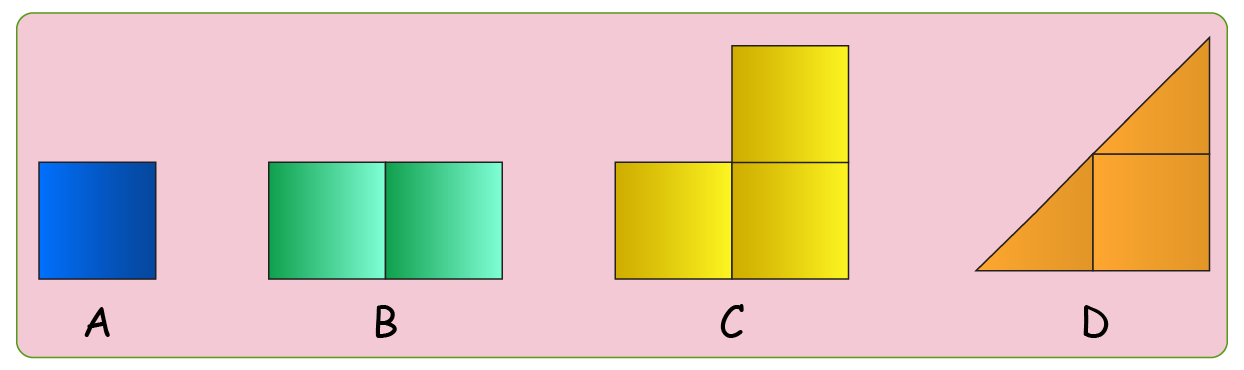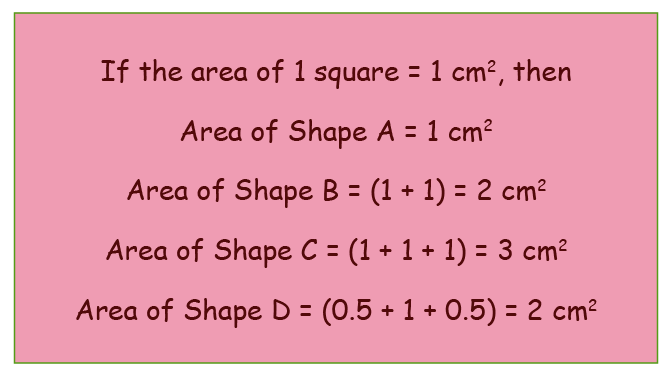Now that you know what an Area is, let's learn how to find the Area of a Triangle, Area of a Quadrilateral, and Area of a Circle.

## Solid Shapes

Solid figures are three-dimensional objects. They have a width, a depth, and a height.

Example: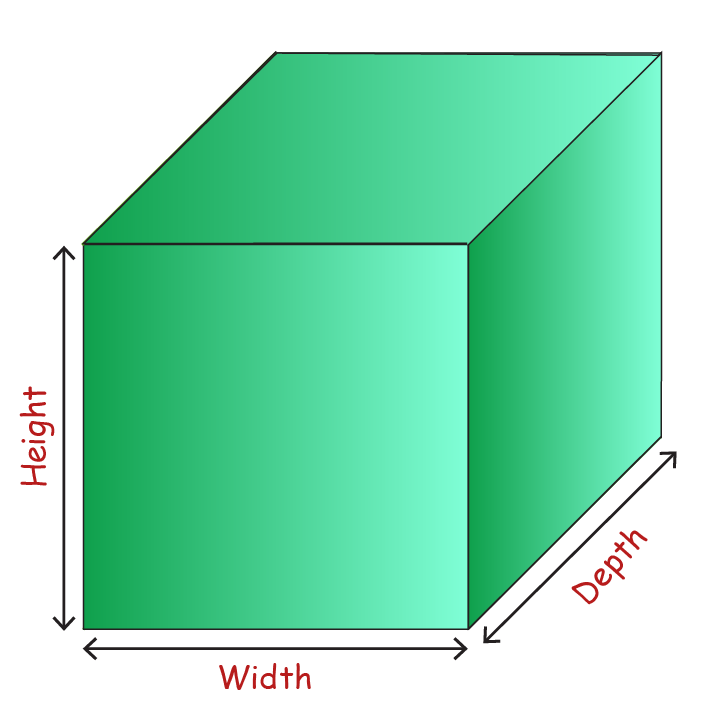We already know Solid Shapes are three dimensional. These 3D objects occupy some space. We will be studying some of the topics like Cuboid Basics, Right Circular Cylinder Basics, Right Circular Cone Basics, and Sphere Basics.

## Surface Area

The surface area of a solid shape with flat faces is the combined area of all its faces.

Example: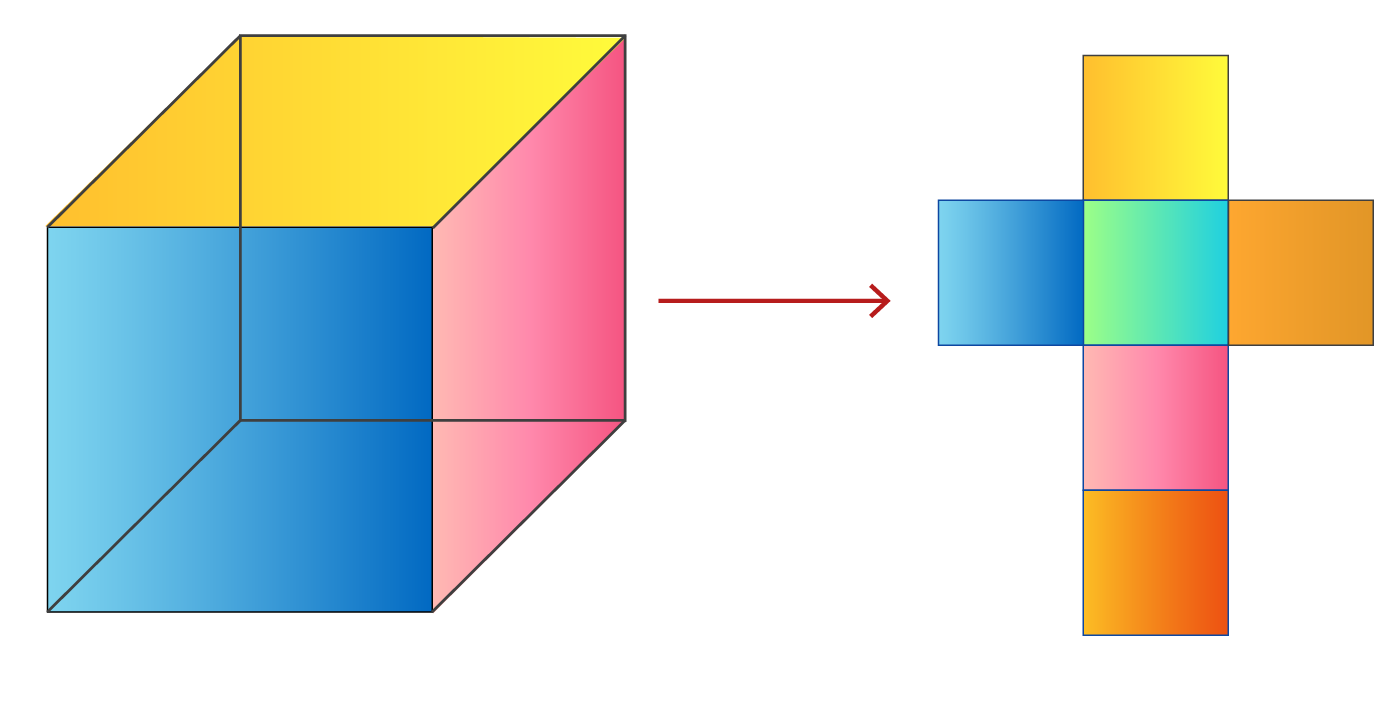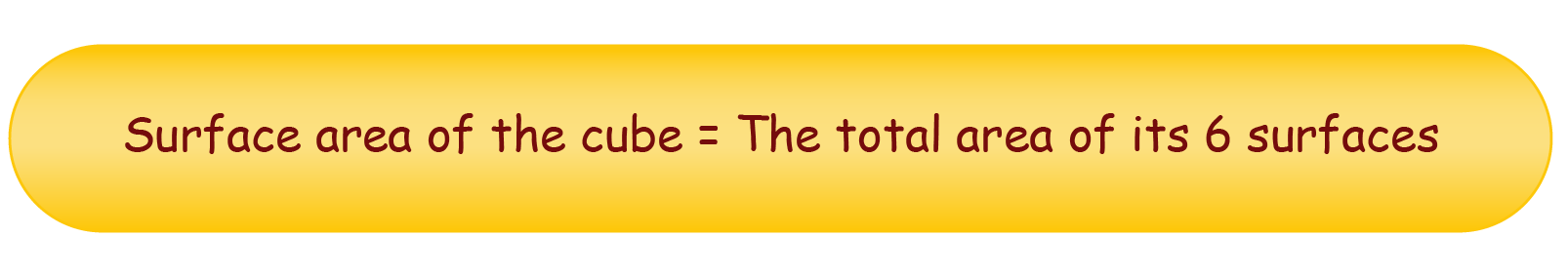In this topic of Surface Area, you will learn how to determine the Surface Area of a Cuboid, Surface Area of Cylinder, Surface Area of Cone, Surface Area of Sphere and Surface Area of Rectangular Prism.

## Volume

Volume is the space enclosed or occupied by any three-dimensional object or solid shape.

Example: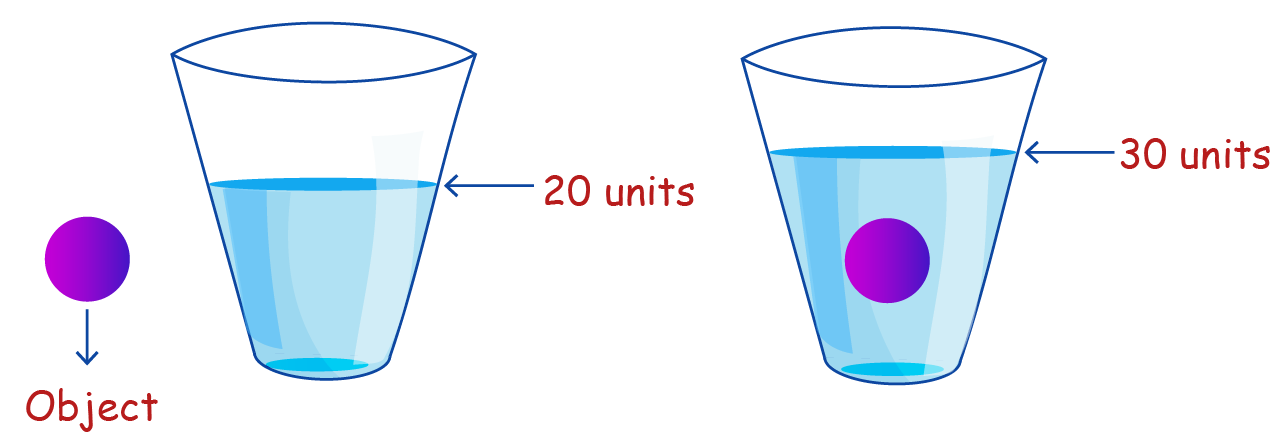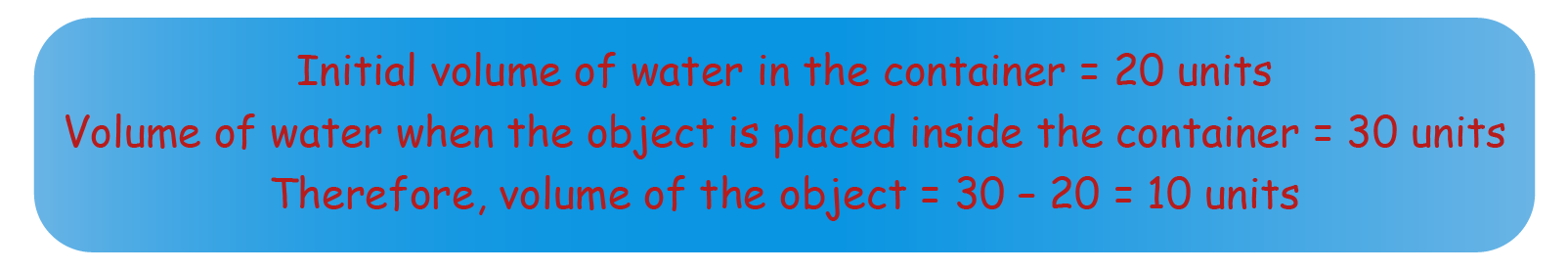Finding the Volume of an object can help us determine the amount required to fill that object. For example, the amount of water in a bottle. We will now learn how to find the Volume of a Cuboid, Volume of a Cylinder, Volume of a Cone, Volume of a Sphere and Volume of a Pyramid.

## Time

Time is the ongoing sequence of events taking place. It is used to quantify the duration of the events. It also helps us set the start time or the end time of events.

Example: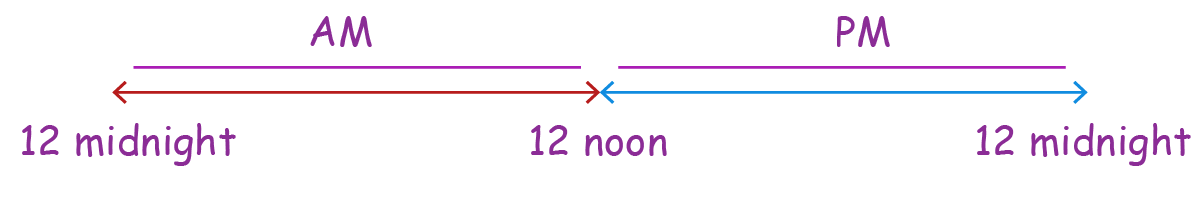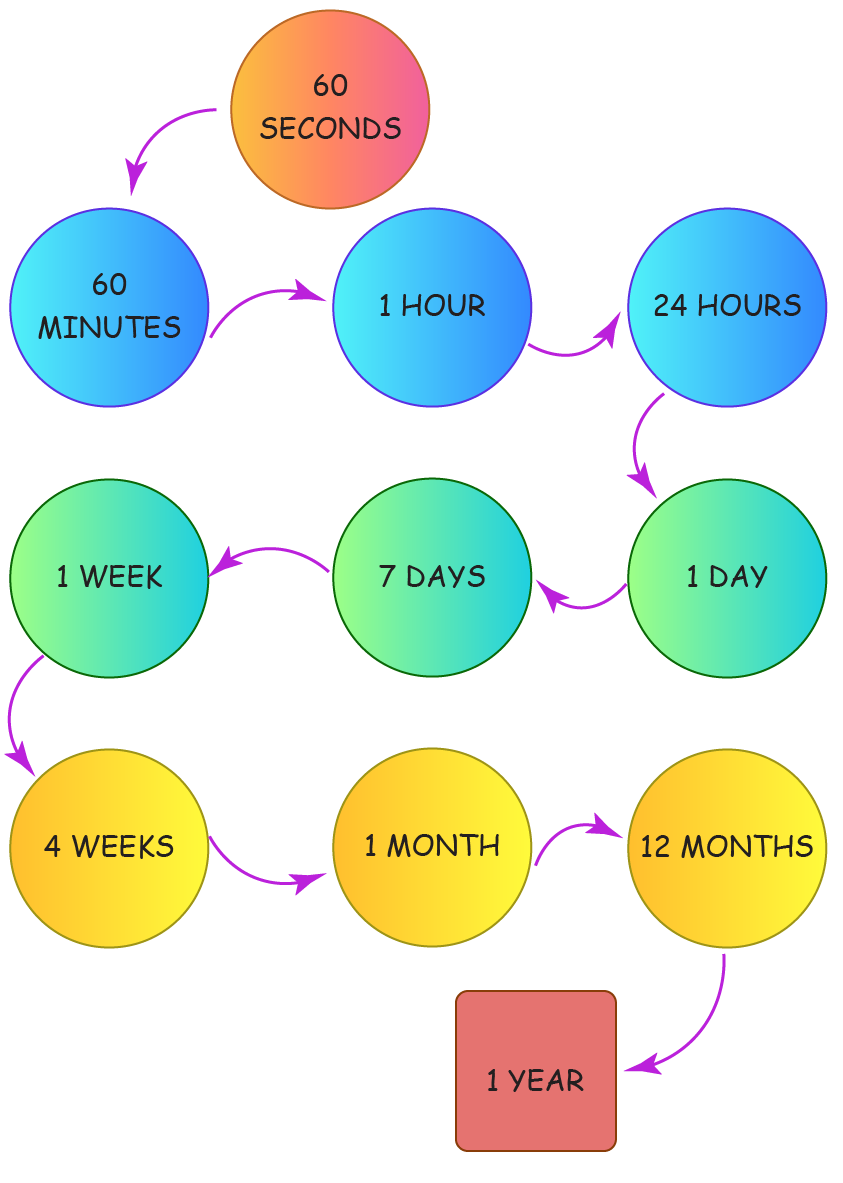One of the very first experiences we have with mathematics is learning how to measure Time. You may already know that the measurement of time is done using a watch and a calendar. Now, let's learn how to Read and Represent Time along with how to Read a Calendar.